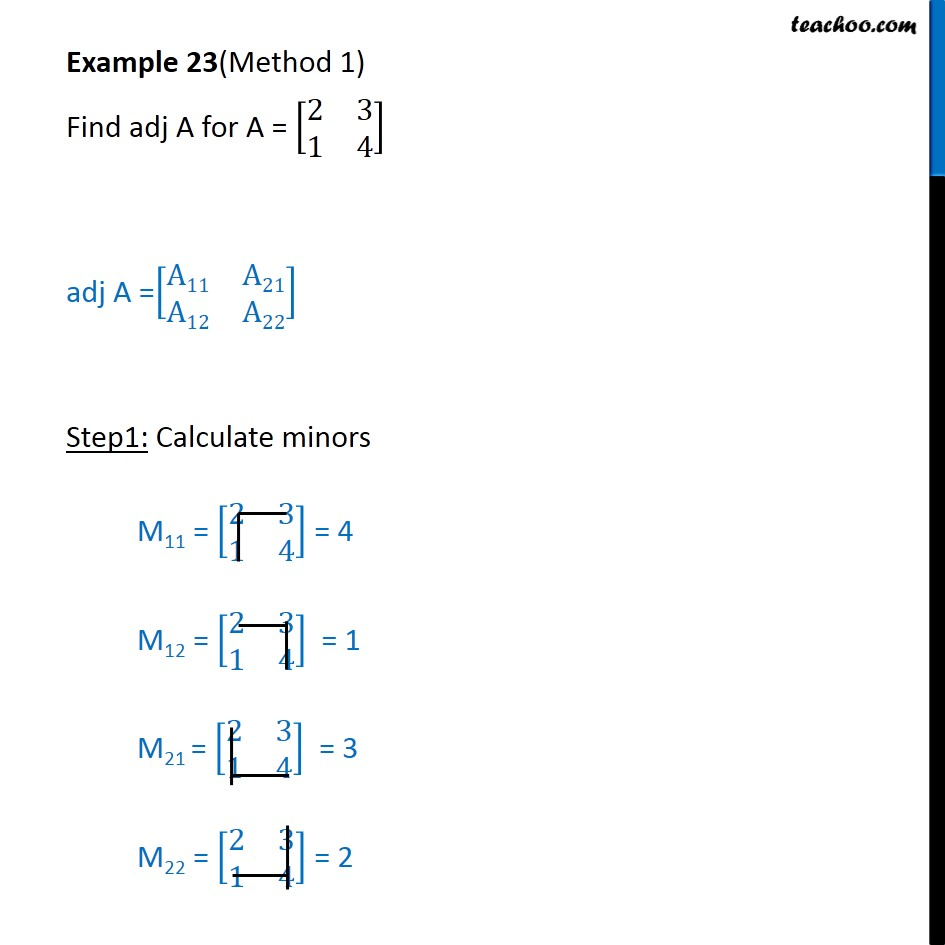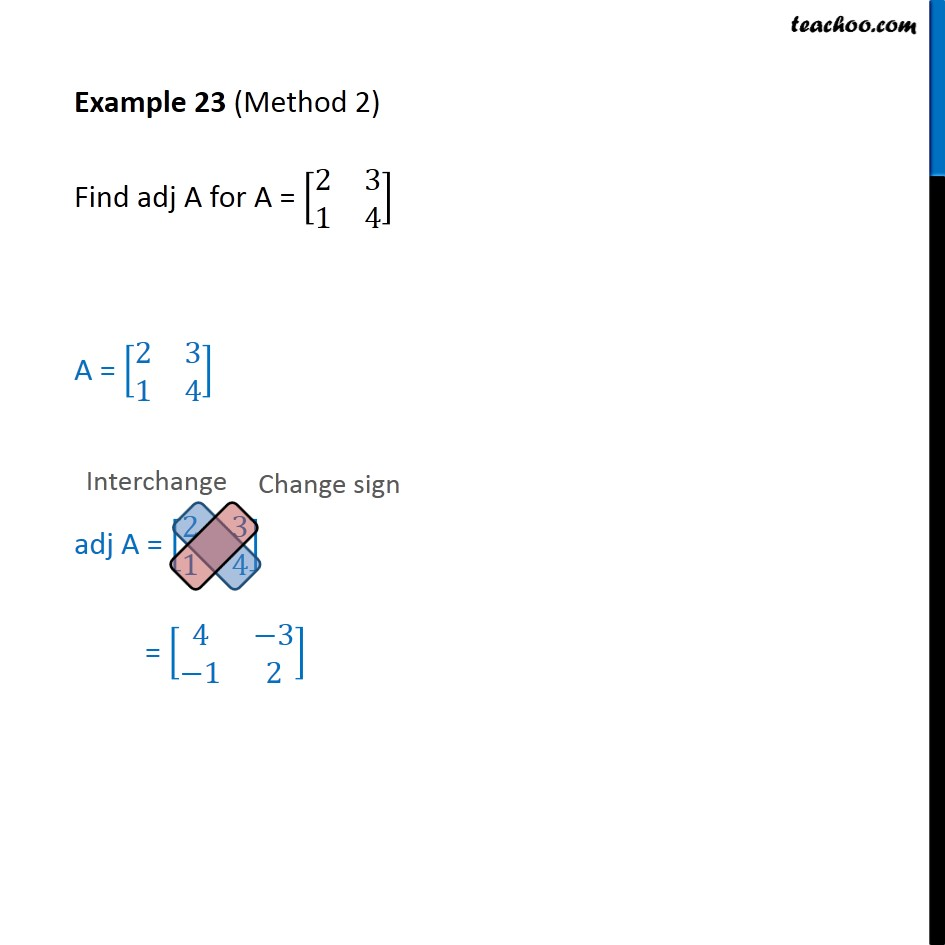Chapter 4 Class 12 Determinants
Concept wiseLearn in your speed, with individual attention - Teachoo Maths 1-on-1 Class

### Transcript

Example 12(Method 1) Find adj A for A = 2﷮3﷮1﷮4﷯﷯ adj A = A11﷮A21﷮A12﷮A22﷯﷯ Step1: Calculate minors M11 = 2﷮3﷮1﷮4﷯﷯ = 4 M12 = 2﷮3﷮1﷮4﷯﷯ = 1 M21 = 2﷮3﷮1﷮4﷯﷯ = 3 M22 = 2﷮3﷮1﷮4﷯﷯ = 2 Step2: Calculate cofactors A11 = ( −1)﷮1 + 1﷯ × 4 = (-1)2 × 4 = 1 × 4 = 4 A12 = ( −1)﷮1+2﷯ M12 = ( −1)﷮3﷯ × (1) = ( −1) (1) = −1 A21 = ( −1)﷮2+1﷯ M21 = ( −1)﷮3﷯ (3) = ( −1) (3) = −3 A22 = ( −1)﷮2+2﷯ . M22 = ( −1)﷮4﷯ . 2 = 2 Step 3: Calculate adjoint adj A = A11﷮A21﷮A12﷮A22﷯﷯ = 4﷮−3﷮−1﷮2﷯﷯ Example 12 (Method 2) Find adj A for A = 2﷮3﷮1﷮4﷯﷯ A = 2﷮3﷮1﷮4﷯﷯ adj A = 2﷮3﷮1﷮4﷯﷯ = 4﷮−3﷮−1﷮2﷯﷯